# HC Verma solutions for Class 11, Class 12 Concepts of Physics Vol. 1 chapter 7 - Circular Motion [Latest edition]

#### Chapters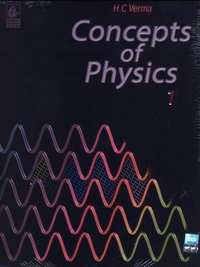## Solutions for Chapter 7: Circular Motion

Below listed, you can find solutions for Chapter 7 of CBSE, Karnataka Board PUC HC Verma for Class 11, Class 12 Concepts of Physics Vol. 1.

Short Answers [Pages 111 - 112]

### HC Verma solutions for Class 11, Class 12 Concepts of Physics Vol. 1 Chapter 7 Circular Motion Short Answers [Pages 111 - 112]

Short Answers | Q 1 | Page 111

You are driving a motorcycle on a horizontal road. It is moving with a uniform velocity. Is it possible to accelerate the motorcycle without putting higher petrol input rate into the engine?

Short Answers | Q 2 | Page 112

Some washing machines have cloth driers. It contains a drum in which wet clothes are kept. As the drum rotates, the water particles get separated from the cloth. The general description of this action is that "the centrifugal force throws the water particles away from the drum". Comment on this statement from the viewpoint of an observer rotating with the drum and the observer who is washing the clothes.

Short Answers | Q 3 | Page 112

A small coin is placed on a record rotating at $33\frac{1}{3}$ rev/minute. The coin does not slip on the record. Where does it get the required centripetal force from ?

Short Answers | Q 4 | Page 112

A bird while flying takes a left turn, where does it get the centripetal force from ?

Short Answers | Q 5 | Page 112

Is it necessary to express all angles in radian while using the equation ω = ω0 + at ?

Short Answers | Q 6 | Page 112

After a good meal at a party you wash your hands and find that you have forgotten to bring your handkerchief. You shake your hand vigorously to remove the water as much as you can. Why is water removed in this process?

Short Answers | Q 7 | Page 112

A smooth block loosely fits in a circular tube placed on a horizontal surface. The block moves in a uniform circular motion along the tube. Which wall (inner or outer) will exert a nonzero normal contact force on the block?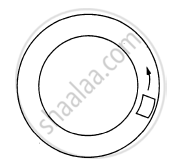Short Answers | Q 8 | Page 112

Consider the circular motion of the earth around the sun. Which of the following statements is more appropriate ?

(a) Gravitational attraction of the sun on the earth is equal to the centripetal force.
(b) Gravitational attraction of the sun on the earth is the centripetal force.

Short Answers | Q 9 | Page 112

A car driver going at some speed v suddenly finds a wide wall at a distance r. Should he apply brakes or turn the car in a circle of radius r to avoid hitting the wall?

Short Answers | Q 10 | Page 112

A heavy mass m is hanging from a string in equilibrium without breaking it. When this same mass is set into oscillation, the string breaks. Explain.

MCQ [Pages 112 - 113]

### HC Verma solutions for Class 11, Class 12 Concepts of Physics Vol. 1 Chapter 7 Circular Motion MCQ [Pages 112 - 113]

MCQ | Q 1 | Page 112

When a particle moves in a circle with a uniform speed

• its velocity and acceleration are both constant

• its velocity is constant but the acceleration changes

• its acceleration is constant but the velocity changes

• its velocity and acceleration both change.

MCQ | Q 2 | Page 112

Tow cars having masses m1 and m2 moves in circles of radii r1 and r2 respectively. If they complete the circle in equal time, the ratio of their angular speed ω12 is

• m1/m

• r1/r

• m1r1/m2/r

• 1.

MCQ | Q 3 | Page 112

A car moves at a constant speed on a road as shown in figure. The normal force by the road on the car NA and NB when it is at the points A and B respectively.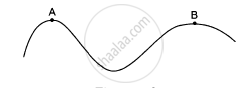• NA = NB

• NA > N

• NA < N

•  insufficient

MCQ | Q 4 | Page 112

A particle of mass m is observed from an inertial frame of reference and is found to move in a circle of radius r with a uniform speed v. The centrifugal force on it is

• $\frac{\text{mv}^2}{\text{r}}$ towards the centre

• $\frac{\text{mv}^2}{\text{r}}$ away from the centre

• $\frac{\text{mv}^2}{\text{r}}$ along the tangent through the particle

•  zero

MCQ | Q 5 | Page 112

A particle of mass m rotates with a uniform angular speed ω. It is viewed from a frame rotating about the Z-axis with a uniform angular speed ω0. The centrifugal force on the particle is

• 2

• $\text{ m } \omega_0^2 \text{ a }$

• $\text{ m } \left( \frac{\omega + \omega_0}{2} \right)^2 \text{a}$

• mω ω0 a.

MCQ | Q 6 | Page 112

A particle is kept fixed on a turntable rotating uniformly. As seen from the ground the particle goes in a circle, its speed is 20 cm/s and acceleration is 20 cm/s2. The particle is now shifted to a new position to make the radius half of the original value. The new value of the speed and acceleration will be

• 10 cm/s, 10 cm/s

• 10 cm/s, 80 cm/s2

• 40 cm/s, 10 cm/s2

• 40 cm/s, 40 cm/s2

MCQ | Q 7 | Page 112

Water in a bucket is whirled in a vertical circle with string attached to it. The water does no fall down even when the bucket is inverted at the top of its path. We conclude that in this position

• $\text{mg }= \frac{\text{mv}^2}{\text{r}}$

• mg is greater than $\frac{\text{mv}^2}{\text{r}}$

• mg is not greater than $\frac{\text{mv}^2}{\text{r}}$

• mg is not less than $\frac{\text{mv}^2}{\text{r}}$

MCQ | Q 8 | Page 113

A stone of mass m tied to a string of length l is rotated in a circle with the other end of the string as the centre. The speed of the stone is v. If the string breaks, the stone will move

• towards the centre

• away from the centre

• along a tangent

• will stop.

MCQ | Q 9 | Page 113

A coin placed on a rotating turntable just slips. If it is placed at a distance of 4 cm from the centre. If the angular velocity of the turntable is doubled, it will just slip at a distance of

• 1 cm

• 2 cm

• 4 cm

•  8 cm

MCQ | Q 10 | Page 113

A motorcycle is going on an overbridge of radius R. The driver maintains a constant speed. As the motorcycle is ascending on the overbridge, the normal force on it

•  increases

• decreases

•  remains the same

• fluctuates.

MCQ | Q 11 | Page 113

Three identical cars A, B and C are moving at the same speed on three bridges. The car A goes on a place bridge, B on a bridge convex upward and C goes on a bridge concave upward. Let FA, FB and FC be the normal forces exerted by the car on the bridges when they are at the middle of bridges.

• FA is maximum of the three forces.

• FB is maximum of the three forces.

• FC is maximum of the three forces.

• FA = FB = FC

MCQ | Q 12 | Page 113

A train A runs from east to west and another train B of the same mass runs from west to east at the same speed along the equator. A presses the track with a force F1 and B presses the track with a force F2

• F1 > F2.

• F1 < F2

• F1 = F2

• the information is insufficient to find the relation between F1 and F2

MCQ | Q 13 | Page 113

If the earth stop rotating, the apparent value of g on its surface will

•  increase everywhere

• decrease everywhere

• remain the same everywhere

• increase at some places and remain the same at some other places.

MCQ | Q 14 | Page 113

A rod of length L is pivoted at one end and is rotated with a uniform angular velocity in a horizontal plane. Let T1 and T2 be the tensions at the points L/4 and 3L/4 away from the pivoted ends.

• T1 > T2

• T2 > T1

• T1 = T2

• The relation between T1 and T2 depends on whether the rod rotates clockwise or anticlockwise.

MCQ | Q 15 | Page 113

A simple pendulum having a bob of mass m is suspended from the ceiling of a car used in a stunt film shooting. the car moves up along an inclined cliff at a speed v and makes a jump to leave the cliff and lands at some distance. Let R be the maximum height of the car from the top of the cliff. The tension in the string when the car is in air is

• mg

• $\text{ mg} - \frac{\text{mv}^2}{\text{R}}$

• $\text{mg} + \frac{\text{mv}^2}{\text{R}}$

• zero.

MCQ | Q 16 | Page 113

Let θ denote the angular displacement of a simple pendulum oscillating in a vertical plane. If the mass of the bob is m, the tension is the string is mg cos θ

• always

• never

• at the extreme positions

• at the mean position.

MCQ [Pages 113 - 114]

### HC Verma solutions for Class 11, Class 12 Concepts of Physics Vol. 1 Chapter 7 Circular Motion MCQ [Pages 113 - 114]

MCQ | Q 1 | Page 113

An object follows a curved path. The following quantities may remain constant during the motion

(a) speed
(b) velocity
(c) acceleration
(d) magnitude of acceleration.

MCQ | Q 2 | Page 113

Assume that the earth goes round the sun in a circular orbit with a constant speed of 30 kms

• The average velocity of the earth from 1st jan, 90 to 30th June, 90 is zero.

• The average acceleration during the above period is 60 km/s2

• The average speed from 1st Jan, 90 to 31st Dec, 90 is zero.

• The instantaneous acceleration of the earth points towards the sun.

MCQ | Q 3 | Page 113

The position vector of a particle in a circular motion about the origin sweeps out equal area in equal time. Its
(a) velocity remains constant
(b) speed remains constant
(c) acceleration remains constant
(d) tangential acceleration remains constant.

MCQ | Q 4 | Page 113

A particle is going in a spiral path as shown in figure with constant speed.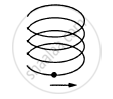• The velocity of the particle is constant.

• The acceleration of the particle is constant.

• The magnitude of acceleration is constant.

• The magnitude of acceleration is decreasing continuously.

MCQ | Q 5 | Page 114

A car of mass M is moving on a horizontal circular path of radius r. At an instant its speed is v and is increasing at a rate a.
(a) The acceleration of the car is towards the centre of the path.
(b) The magnitude of the frictional force on the car is greater than $\frac{\text{mv}^2}{\text{r}}$

(c) The friction coefficient between the ground and the car is not less than a/g.
(d) The friction coefficient between the ground and the car is $\mu = \tan^{- 1} \frac{\text{v}^2}{\text{rg}.}$

MCQ | Q 6 | Page 114

A circular road of radius r is banked for a speed v = 40 km/hr. A car of mass of m attempts to go on the circular road. The friction coefficient between the tyre and the road is negligible.

(a) The are cannot make a turn without skidding.
(b) If the car turns at a speed less than 40 km/hr, it will slip down.

(c) If the car turns at the car is equal to $\frac{\text{mv}^2}{\text{r}}$

(d) If the car turns at the correct speed of 40 km/hr, the force by the road on the car is greater than mg as well as greater than $\frac{\text{mv}^2}{\text{r}}$

MCQ | Q 7 | Page 114

A person applies constant force $\overrightarrow{\text{F}}$on a particle of mass m and finds that the particle moves in a circle of radius r with a uniform speed v as seen from an inertial frame of reference.

(a) This is not possible.
(b) There are other forces on the particle.
(c) The resultant of the other forces is $\frac{\text{mv}^2}{\text{r}}$ towards the centre.

d) The resultant of the other forces varies in magnitude as well as in direction

Exercise [Pages 114 - 116]

### HC Verma solutions for Class 11, Class 12 Concepts of Physics Vol. 1 Chapter 7 Circular Motion Exercise [Pages 114 - 116]

Exercise | Q 1 | Page 114

Find the acceleration of the moon with respect to the earth from the following data : Distance between the earth and the moon = 3.85 × 105 km and the time taken by the moon to complete one revolution around the earth = 27.3 days.

Exercise | Q 2 | Page 114

Find the acceleration of a particle placed on the surface of the earth at the equator due to earth's rotation. The diameter of earth = 12800 km and it takes 24 hours for the earth to complete one revolution about its axis.

Exercise | Q 3 | Page 114

A particle moves in a circle of radius 1.0 cm at a speed given by v = 2.0 t where v is cm/s and t in seconds.
(a) Find the radial acceleration of the particle at t = 1 s.
(b) Find the tangential acceleration at t = 1 s.
(c) Find the magnitude of the acceleration at t = 1 s.

Exercise | Q 4 | Page 114

A scooter weighing 150 kg together with its rider moving at 36 km/hr is to take a turn of a radius 30 m. What horizontal force on the scooter is needed to make the turn possible ?

Exercise | Q 5 | Page 114

If the horizontal force needed for the turn in the previous problem is to be supplied by the normal force by the road, what should be the proper angle of banking?

Exercise | Q 6 | Page 114

A park has a radius of 10 m. If a vehicle goes round it at an average speed of 18 km/hr, what should be the proper angle of banking?

Exercise | Q 7 | Page 114

If the road of the previous problem is horizontal (no banking), what should be the minimum friction coefficient so that scooter going at 18 km/hr does not skid?

Exercise | Q 8 | Page 114

A circular road of radius 50 m has the angle of banking equal to 30°. At what speed should a vehicle go on this road so that the friction is not used?

Exercise | Q 9 | Page 114

In the Bohr model of hydrogen atom, the electron is treated as a particle going in a circle with the centre at the proton. The proton itself is assumed to be fixed in an inertial frame. The centripetal force is provided by the Coulomb attraction. In the ground state, the electron goes round the proton in a circle of radius 5.3 × 10−11 m. Find the speed of the electron in the ground state. Mass of the electron = 9.1 × 10−31 kg and charge of the electron = 1.6 × 10−19 C.

Exercise | Q 10 | Page 114

A stone is fastened to one end of a string and is whirled in a vertical circle of radius R. Find the minimum speed the stone can have at the highest point of the circle.

Exercise | Q 11 | Page 114

A ceiling fan has a diameter (of the circle through the outer edges of the three blades) of 120 cm and rpm 1500 at full speed. Consider a particle of mass 1 g sticking at the outer end of a blade. How much force does it experience when the fan runs at full speed? Who exerts this force on the particle? How much force does the particle exert on the blade along its surface?

Exercise | Q 12 | Page 114

A mosquito is sitting on an L.P. record disc rotating on a turn table at $33\frac{1}{3}$ revolutions per minute. The distance of the mosquito from the centre of the turn table is 10 cm. Show that the friction coefficient between the record and the mosquito is greater than π2/81. Take g =10 m/s2.

Exercise | Q 13 | Page 114

A simple pendulum is suspended from the ceiling of a car taking a turn of radius 10 m at a speed of 36 km/h. Find the angle made by he string of the pendulum with the vertical if this angle does not change during the turn. Take g = 10 m/s2.

Exercise | Q 14 | Page 115

The bob of a simple pendulum of length 1 m has mass 100 g and a speed of 1.4 m/s at the lowest point in its path. Find the tension in the string at this instant.

Exercise | Q 15 | Page 115

Suppose the bob of the previous problem has a speed of 1.4 m/s when the string makes an angle of 0.20 radian with the vertical. Find the tension at this instant. You can use cos θ ≈ 1 − θ2/2 and SINθ ≈ θ for small θ.

Exercise | Q 16 | Page 115

Suppose the amplitude of a simple pendulum having a bob of mass m is θ0. Find the tension in the string when the bob is at its extreme position.

Exercise | Q 17.1 | Page 115

A person stands on a spring balance at the equator. By what fraction is the balance reading less than his true weight?

Exercise | Q 17.2 | Page 115

A person stands on a spring balance at the equator. If the speed of earth's rotation is increased by such an amount that the balance reading is half the true weight, what will be the length of the day in this case?

Exercise | Q 18 | Page 115

A turn of radius 20 m is banked for the vehicles going at a speed of 36 km/h. If the coefficient of static friction between the road and the tyre is 0.4, what are the possible speeds of a vehicle so that it neither slips down nor skids up?

Exercise | Q 19 | Page 115

A motorcycle has to move with a constant speed on an over bridge which is in the form of a circular arc of radius R and has a total length L. Suppose the motorcycle starts from the highest point.(a) What can its maximum velocity be for which the contact with the road is not broken at the highest point? (b) If the motorcycle goes at speed 1/√2 times the maximum found in part (a), where will it lose the contact with the road? (c) What maximum uniform speed can it maintain on the bridge if it does not lose contact anywhere on the bridge?

Exercise | Q 20 | Page 115

A car goes on a horizontal circular road of radius R, the speed increasing at a constant rate $\frac{\text{dv}}{\text{dt}} = a$ . The friction coefficient between the road and the tyre is μ. Find the speed at which the car will skid.

Exercise | Q 21 | Page 115

A block of mass m is kept on a horizontal ruler. The friction coefficient between the ruler and the block is μ. The ruler is fixed at one end and the block is at a distance L from the fixed end. The ruler is rotated about the fixed end in the horizontal plane through the fixed end. (a) What can the maximum angular speed be for which the block does not slip? (b) If the angular speed of the ruler is uniformly increased from zero at an angular acceleration α, at what angular speed will the block slip?

Exercise | Q 22 | Page 115

A track consists of two circular parts ABC and CDE of equal radius 100 m and  joined smoothly as shown in figure. Each part subtends a right angle at its centre. A cycle weighing 100 kg together with the rider travels at a constant speed of 18 km/h on the track. (a) Find the normal contact force by the road on the cycle when it is at B and at D. (b) Find the force of friction exerted by the track on the tyres when the cycle is at B, C and. (c) Find the normal force between the road and the cycle just before and just after the cycle crosses C. (d) What should be the minimum friction coefficient between the road and the tyre, which will ensure that the cyclist can move with constant speed? Take g = 10 m/s2.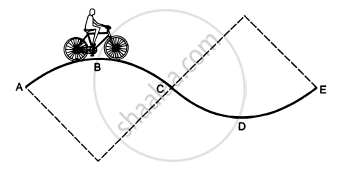Exercise | Q 23 | Page 115

In a children's park a heavy rod is pivoted at the centre and is made to rotate about the pivot so that the rod always remains horizontal. Two kids hold the rod near the ends and thus rotate with the rod (In the following figure). Let the mass of each kid be 15 kg, the distance between the points of the rod where the two kids hold it be 3.0 m and suppose that the rod rotates at the rate of 20 revolutions per minute. Find the force of friction exerted by the rod on one of the kids.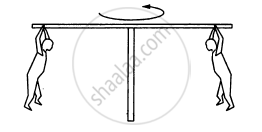Exercise | Q 24 | Page 115

A hemispherical bowl of radius R is rotated about its axis of symmetry which is kept vertical. A  small block is kept in the bowl at a position where the radius makes an angle θ with the vertical. The  block rotates with the bowl without any slipping. The friction coefficient between the block and the bowl surface is μ. Find the range of the angular speed for which the block will not slip.

Exercise | Q 25 | Page 115

A particle is projected with a speed u at an angle θ with the horizontal. Consider a small part of its path near the highest position and take it approximately to be a circular arc. What is the radius of this circular circle? This radius is called the radius of curvature of the curve at the point.

Exercise | Q 26 | Page 115

What is the radius of curvature of the parabola traced out by the projectile in the previous problem at a point where the particle velocity makes an angle θ/2 with the horizontal?

Exercise | Q 27 | Page 115

A block of mass m moves on a horizontal circle against the wall of a cylindrical room of radius R. The floor of the room on which the block moves is smooth but the friction coefficient between the wall and the block is μ. The block is given an initial speed v0. As a function of the speed v writes
(a) the normal force by the wall on the block,
(b) the frictional force by a wall, and
(c) the tangential acceleration of the block.
(d) Integrate the tangential acceleration $\left( \frac{dv}{dt} = v\frac{dv}{ds} \right)$ to obtain the speed of the block after one revolution.

Exercise | Q 28 | Page 116

A table with smooth horizontal surface is fixed in a cabin that rotates with a uniform angular  velocity ω in a circular path of radius R (In the following figure). A smooth groove AB of length L(<<R) is made the surface of the table. The groove makes an angle θ with the radius OA of the circle in which the cabin rotates. A small particle is kept at the point A in the groove and is released to move at the point A in the groove and is released to move along AB. Find the time taken by the particle to reach the point B.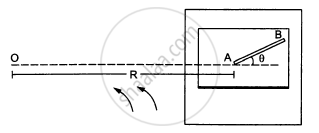Exercise | Q 29 | Page 116

A car moving at a speed of 36 km/hr is taking a turn on a circular road of radius 50 m. A small wooden plate is kept on the seat with its plane perpendicular to the radius of the circular road (In the following figure). A small block of mass 100 g is kept on the seat which rests against the plate. the friction coefficient between the block and the plate is. (a) Find the normal contact force exerted by the plate on the block. (b) The plate is slowly turned so that the angle between the normal to the plate and the radius of the road slowly increases. Find the angle at which the block will just start sliding on the plate.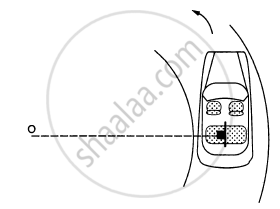Exercise | Q 30 | Page 116

A table with smooth horizontal surface is placed in a circle of a large radius R (In the following figure). A smooth pulley of small radius is fastened to the table. Two masses m and 2m placed on the table are connected through a string going over the pulley. Initially the masses are held by a person with the string along the outward radius and then the  system is released from rest (with respect to the cabin). Find the magnitude of the initial acceleration of the masses as seen from the cabin and the tension in the string.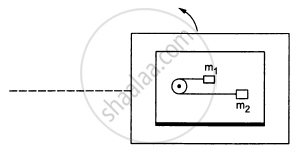## Solutions for Chapter 7: Circular Motion## HC Verma solutions for Class 11, Class 12 Concepts of Physics Vol. 1 chapter 7 - Circular Motion

Shaalaa.com has the CBSE, Karnataka Board PUC Mathematics Class 11, Class 12 Concepts of Physics Vol. 1 CBSE, Karnataka Board PUC solutions in a manner that help students grasp basic concepts better and faster. The detailed, step-by-step solutions will help you understand the concepts better and clarify any confusion. HC Verma solutions for Mathematics Class 11, Class 12 Concepts of Physics Vol. 1 CBSE, Karnataka Board PUC 7 (Circular Motion) include all questions with answers and detailed explanations. This will clear students' doubts about questions and improve their application skills while preparing for board exams.

Further, we at Shaalaa.com provide such solutions so students can prepare for written exams. HC Verma textbook solutions can be a core help for self-study and provide excellent self-help guidance for students.

Concepts covered in Class 11, Class 12 Concepts of Physics Vol. 1 chapter 7 Circular Motion are Lubrication - (Laws of Motion), Law of Conservation of Linear Momentum and Its Applications, Aristotle’s Fallacy, The Law of Inertia, Newton’s Second Law of Motion, Newton's Third Law of Motion, Equilibrium of a Particle, Common Forces in Mechanics, Circular Motion and Its Characteristics, Solving Problems in Mechanics, Static and Kinetic Friction, Laws of Friction, Inertia, Intuitive Concept of Force, Dynamics of Uniform Circular Motion - Centripetal Force, Examples of Circular Motion (Vehicle on a Level Circular Road, Vehicle on a Banked Road), Newton's First Law of Motion, Rolling Friction, Introduction of Motion in One Dimension, Conservation of Momentum.

Using HC Verma Class 11, Class 12 Concepts of Physics Vol. 1 solutions Circular Motion exercise by students is an easy way to prepare for the exams, as they involve solutions arranged chapter-wise and also page-wise. The questions involved in HC Verma Solutions are essential questions that can be asked in the final exam. Maximum CBSE, Karnataka Board PUC Class 11, Class 12 Concepts of Physics Vol. 1 students prefer HC Verma Textbook Solutions to score more in exams.

Get the free view of Chapter 7, Circular Motion Class 11, Class 12 Concepts of Physics Vol. 1 additional questions for Mathematics Class 11, Class 12 Concepts of Physics Vol. 1 CBSE, Karnataka Board PUC, and you can use Shaalaa.com to keep it handy for your exam preparation.